Home
Hostname: page-component-568f69f84b-xr9nb Total loading time: 0.175 Render date: 2021-09-21T02:07:51.073Z Has data issue: true Feature Flags: { "shouldUseShareProductTool": true, "shouldUseHypothesis": true, "isUnsiloEnabled": true, "metricsAbstractViews": false, "figures": true, "newCiteModal": false, "newCitedByModal": true, "newEcommerce": true, "newUsageEvents": true }Compositio Mathematica

# Local cohomology of Du Bois singularities and applications to families

Published online by Cambridge University Press:  27 July 2017

## Abstract

In this paper we study the local cohomology modules of Du Bois singularities. Let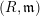$(R,\mathfrak{m})$ be a local ring; we prove that if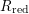$R_{\text{red}}$ is Du Bois, then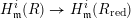$H_{\mathfrak{m}}^{i}(R)\rightarrow H_{\mathfrak{m}}^{i}(R_{\text{red}})$ is surjective for every$i$ . We find many applications of this result. For example, we answer a question of Kovács and Schwede [Inversion of adjunction for rational and Du Bois pairs, Algebra Number Theory 10 (2016), 969–1000; MR 3531359] on the Cohen–Macaulay property of Du Bois singularities. We obtain results on the injectivity of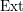$\operatorname{Ext}$ that provide substantial partial answers to questions in Eisenbud et al. [Cohomology on toric varieties and local cohomology with monomial supports, J. Symbolic Comput. 29 (2000), 583–600] in characteristic$0$ . These results can also be viewed as generalizations of the Kodaira vanishing theorem for Cohen–Macaulay Du Bois varieties. We prove results on the set-theoretic Cohen–Macaulayness of the defining ideal of Du Bois singularities, which are characteristic-$0$ analogs and generalizations of results of Singh–Walther and answer some of their questions in Singh and Walther [On the arithmetic rank of certain Segre products, in Commutative algebra and algebraic geometry, Contemporary Mathematics, vol. 390 (American Mathematical Society, Providence, RI, 2005), 147–155]. We extend results on the relation between Koszul cohomology and local cohomology for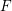$F$ -injective and Du Bois singularities first shown in Hochster and Roberts [The purity of the Frobenius and local cohomology, Adv. Math. 21 (1976), 117–172; MR 0417172 (54 #5230)]. We also prove that singularities of dense$F$ -injective type deform.

## MSC classification

Type
Research Article
Information
Compositio Mathematica , October 2017 , pp. 2147 - 2170

## Access options

Get access to the full version of this content by using one of the access options below. (Log in options will check for institutional or personal access. Content may require purchase if you do not have access.)

## References

Bhatt, B., On the non-existence of small Cohen–Macaulay algebras , J. Algebra 411 (2014), 111.CrossRefGoogle Scholar
Bhatt, B., Schwede, K. and Takagi, S., The weak ordinarity conjecture and$F$ -singularities, Adv. Stud. Pure Math. (2013), to appear, arXiv:1307.3763.Google Scholar
Bourbaki, N., Commutative algebra , inElements of mathematics (Berlin) (Springer, Berlin, 1998), chs. 1–7, translated from the French, reprint of the 1989 English translation;MR 1727221 (2001g:13001).Google Scholar
Brodmann, M. P. and Sharp, R. Y., Local cohomology: an algebraic introduction with geometric applications, Cambridge Studies in Advanced Mathematics, vol. 60 (Cambridge University Press, Cambridge, 1998); MR 1613627 (99h:13020).CrossRefGoogle Scholar
Cortiñas, G., Haesemeyer, C., Walker, M. E. and Weibel, C., A negative answer to a question of Bass , Proc. Amer. Math. Soc. 139 (2011), 11871200; MR 2748413.CrossRefGoogle Scholar
de Fernex, T., Ein, L. and Mustaţă, M., Vanishing theorems and singularities in birational geometry, Preprint (2014), http://www.math.uic.edu/∼ein/DFEM.pdf.Google Scholar
De Stefani, A. and Núñez-Betancourt, L., F-threshold of graded rings , Nagoya Math. J. (2016), 128, doi:10.1017/nmj.2016.65.CrossRefGoogle Scholar
Deligne, P., Théorie de Hodge. III , Publ. Math. Inst. Hautes Études Sci. 44 (1974), 577; MR 0498552 (58 #16653b).CrossRefGoogle Scholar
Demazure, M., Anneaux gradués normaux. Introduction à la théorie des singularités, II , Travaux en Cours, vol. 37 (Hermann, Paris, 1988), 3568; MR 1074589 (91k:14004).Google Scholar
Du Bois, P., Complexe de de Rham filtré d’une variété singulière , Bull. Soc. Math. France 109 (1981), 4181; MR 613848 (82j:14006).CrossRefGoogle Scholar
Dwyer, W., Greenlees, J. P. C. and Iyengar, S., Finiteness in derived categories of local rings , Comment. Math. Helv. 81 (2006), 383432; MR 2225632.CrossRefGoogle Scholar
Eisenbud, D., Mustaţă, M. and Stillman, M., Cohomology on toric varieties and local cohomology with monomial supports , J. Symbolic Comput. 29 (2000), 583600.CrossRefGoogle Scholar
Enescu, F. and Hochster, M., The Frobenius structure of local cohomology , Algebra Number Theory 2 (2008), 721754; MR 2460693 (2009i:13009).CrossRefGoogle Scholar
Fedder, R., F-purity and rational singularity , Trans. Amer. Math. Soc. 278 (1983), 461480; MR 701505 (84h:13031).Google Scholar
Fedder, R. and Watanabe, K., A characterization of F-regularity in terms of F-purity , in Commutative algebra (Berkeley, CA, 1987), Mathematical Sciences Research Institute Publications, vol. 15 (Springer, New York, 1989), 227245; MR 1015520 (91k:13009).CrossRefGoogle Scholar
Graf, P. and Kovács, S. J., Potentially Du Bois spaces , J. Singul. 8 (2014), 117134; MR 3395242.Google Scholar
Hara, N. and Watanabe, K.-I., F-regular and F-pure rings vs. log terminal and log canonical singularities , J. Algebraic Geom. 11 (2002), 363392; MR 1874118 (2002k:13009).CrossRefGoogle Scholar
Hochster, M. and Huneke, C., Tight closure in equal characteristic zero, Preprint (2006),http://www.math.lsa.umich.edu/∼hochster/tcz.ps.Google Scholar
Hochster, M. and Roberts, J. L., The purity of the Frobenius and local cohomology , Adv. Math. 21 (1976), 117172; MR 0417172 (54 #5230).CrossRefGoogle Scholar
Horiuchi, J., Miller, L. E. and Shimomoto, K., Deformation of F-injectivity and local cohomology , Indiana Univ. Math. J. 63 (2014), 11391157; with an appendix by Karl Schwede and Anurag K. Singh; MR 3263925.CrossRefGoogle Scholar
Huber, A. and Jörder, C., Differential forms in the h-topology , Algebr. Geom. 1 (2014), 449478; MR 3272910.CrossRefGoogle Scholar
Huneke, C. and Lyubeznik, G., On the vanishing of local cohomology modules , Invent. Math. 102 (1990), 7393.CrossRefGoogle Scholar
Kollár, J., Singularities of the minimal model program, Cambridge Tracts in Mathematics, vol. 200 (Cambridge University Press, Cambridge, 2013), with the collaboration of Sándor Kovács; MR 3057950.CrossRefGoogle Scholar
Kollár, J. and Kovács, S. J., Log canonical singularities are Du Bois , J. Amer. Math. Soc. 23 (2010), 791813; MR 2629988.CrossRefGoogle Scholar
Kollár, J. and Mori, S., Birational geometry of algebraic varieties, Cambridge Tracts in Mathematics, vol. 134 (Cambridge University Press, Cambridge, 1998), with the collaboration of C. H. Clemens and A. Corti, translated from the 1998 Japanese original; MR 1658959 (2000b:14018).CrossRefGoogle Scholar
Kovács, S. J., Rational, log canonical, Du Bois singularities: on the conjectures of Kollár and Steenbrink , Compositio Math. 118 (1999), 123133; MR 1713307 (2001g:14022).CrossRefGoogle Scholar
Kovács, S. J., Du Bois pairs and vanishing theorems , Kyoto J. Math. 51 (2011), 4769; MR 2784747.CrossRefGoogle Scholar
Kovács, S. J. and Schwede, K., Hodge theory meets the minimal model program: a survey of log canonical and Du Bois singularities , in Topology of stratified spaces, Mathematical Sciences Research Institute Publications, vol. 58, eds Friedman, G., Hunsicker, E., Libgober, A. and Maxim, L. (Cambridge University Press, Cambridge, 2011), 5194.Google Scholar
Kovács, S. J. and Schwede, K., Du Bois singularities deform , in Minimal models and extremal rays, Advanced Studies in Pure Mathematics, vol. 70 (Mathematics Society of Japan, Tokyo, 2016), 4966.Google Scholar
Kovács, S. J. and Schwede, K., Inversion of adjunction for rational and Du Bois pairs , Algebra Number Theory 10 (2016), 9691000; MR 3531359.CrossRefGoogle Scholar
Kurano, K., Sato, E.-I., Singh, A. K. and Watanabe, K.-I., Multigraded rings, diagonal subalgebras, and rational singularities , J. Algebra 332 (2009), 32483267.CrossRefGoogle Scholar
Lee, B., Local acyclic fibrations and the de Rham complex , Homology, Homotopy Appl. 11 (2009), 115140; MR 2506129.CrossRefGoogle Scholar
Lyubeznik, G., On the vanishing of local cohomology in characteristic p > 0 , Compositio Math. 142 (2006), 207221.CrossRefGoogle Scholar
Ma, L., Finiteness properties of local cohomology for F-pure local rings , Int. Math. Res. Not. IMRN 2014 (2014), 54895509.CrossRefGoogle Scholar
Ma, L., F-injectivity and Buchsbaum singularities , Math. Ann. 362 (2015), 2542.CrossRefGoogle Scholar
Ma, L. and Zhang, W., Eulerian graded D-modules , Math. Res. Lett. 21 (2014), 149167.CrossRefGoogle Scholar
Mustaţă, M. and Srinivas, V., Ordinary varieties and the comparison between multiplier ideals and test ideals , Nagoya Math. J. 204 (2011), 125157; MR 2863367.CrossRefGoogle Scholar
Patakfalvi, Z., Semi-negativity of Hodge bundles associated to Du Bois families , J. Pure Appl. Algebra 219 (2015), 53875393; MR 3390027.CrossRefGoogle Scholar
Puthenpurakal, T. J., De Rham cohomology of local cohomology modules: the graded case , Nagoya Math. J. 217 (2015), 121.CrossRefGoogle Scholar
Saito, M., Mixed Hodge complexes on algebraic varieties , Math. Ann. 316 (2000), 283331; MR 1741272 (2002h:14012).CrossRefGoogle Scholar
Schenzel, P., Applications of dualizing complexes to Buchsbaum rings , Adv. Math. 44 (1982), 6177; MR 654548 (83j:13011).CrossRefGoogle Scholar
Schwede, K., A simple characterization of Du Bois singularities , Compositio Math. 143 (2007), 813828; MR 2339829.CrossRefGoogle Scholar
Schwede, K., F-injective singularities are Du Bois , Amer. J. Math. 131 (2009), 445473; MR 2503989.CrossRefGoogle Scholar
Singh, A. K. and Walther, U., On the arithmetic rank of certain Segre products , in Commutative algebra and algebraic geometry, Contemporary Mathematics, vol. 390 (American Mathematical Society, Providence, RI, 2005), 147155.CrossRefGoogle Scholar
Singh, A. K. and Walther, U., Local cohomology and pure morphisms , Illinois J. Math. 51 (2007), 287298.Google Scholar
Steenbrink, J. H. M., Cohomologically insignificant degenerations , Compositio Math. 42 (1980–1981), 315320; MR 607373 (84g:14011).Google Scholar
Varbaro, M., Cohomological and projective dimensions , Compositio Math. 149 (2013), 12031210; MR 3078644.CrossRefGoogle Scholar
Watanabe, K.-I., Some remarks concerning Demazure’s construction of normal graded rings , Nagoya Math J. 83 (1981), 203221.CrossRefGoogle Scholar
5
Cited by

# Send article to Kindle

Note you can select to send to either the @free.kindle.com or @kindle.com variations. ‘@free.kindle.com’ emails are free but can only be sent to your device when it is connected to wi-fi. ‘@kindle.com’ emails can be delivered even when you are not connected to wi-fi, but note that service fees apply.

Find out more about the Kindle Personal Document Service.

Local cohomology of Du Bois singularities and applications to families
Available formats
×

# Send article to Dropbox

To send this article to your Dropbox account, please select one or more formats and confirm that you agree to abide by our usage policies. If this is the first time you use this feature, you will be asked to authorise Cambridge Core to connect with your <service> account. Find out more about sending content to Dropbox.

Local cohomology of Du Bois singularities and applications to families
Available formats
×

# Send article to Google Drive

To send this article to your Google Drive account, please select one or more formats and confirm that you agree to abide by our usage policies. If this is the first time you use this feature, you will be asked to authorise Cambridge Core to connect with your <service> account. Find out more about sending content to Google Drive.

Local cohomology of Du Bois singularities and applications to families
Available formats
×
×